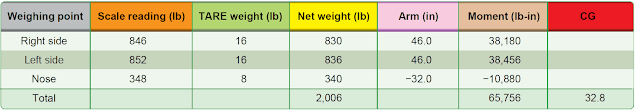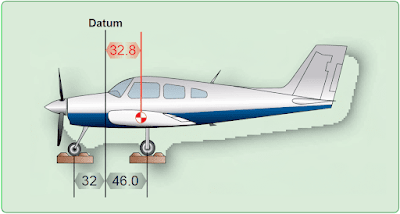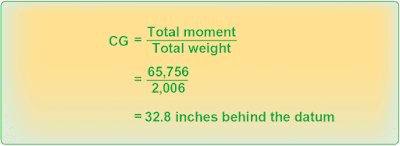# Determining the CG

When the aircraft is in its level flight attitude, drop a plumb line from the datum and make a mark on the hangar floor below the tip of the bob. Draw a chalk line through this point parallel to the longitudinal axis of the aircraft.

Then, draw lateral lines between the actual weighing points for the main wheels, and make a mark along the longitudinal line at the weighing point for the nosewheel or the tailwheel. These lines and marks on the floor allow accurate measurements between the datum and the weighting points to determine their arms.

Determine the CG by adding the weight and moment of each weighing point to determine the total weight and total moment. Then, divide the total moment by the total weight to determine the CG relative to the datum. As an example of locating the CG with respect to the datum, which in this case is the firewall, consider the tricycle landing gear airplane as detailed in the Figure 1 table and illustrated in Figure 2.Figure 1. Locating the CG of an airplane relative to the datumFigure 2. The datum is located at the firewall

When the airplane is on the scales with the parking brakes off, place chocks around the wheels to keep the airplane from rolling. Subtract the weight of the chocks, called tare weight, from the scale reading to determine the net weight at each weighing point. Multiply each net weight by its arm to determine its moment, and then determine the total weight and total moment. The CG is determined by dividing the total moment by the total weight. [Figure 3]Figure 3. Determining the CG

The airplane illustrated in Figures 1 and 2 has a net weight of 2,006 pounds, and its CG is 32.8 inches behind the datum.

RELATED POSTS